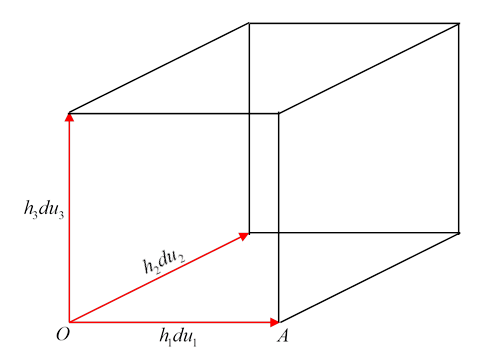### Div, Grad and Curl in Orthogonal Curvilinear Coordinates

The treatment here is standard, following that in Abraham and Becker, Classical Theory of Electricity and Magnetism.

Problems with a particular symmetry, such as cylindrical or spherical, are best attacked using coordinate systems that take full advantage of that symmetry.  For example, the Schrödinger equation for the hydrogen atom is best solved using spherical polar coordinates.  For this and other differential equation problems, then, we need to find the expressions for differential operators in terms of the appropriate coordinates.

We only look at orthogonal coordinate systems, so that locally the three axes (such as $r,θ,φ$ ) are a mutually perpendicular set.

We denote the curvilinear coordinates by $( u 1 , u 2 , u 3 ).$ The standard Cartesian coordinates for the same space are as usual $( x,y,z ).$

Suppose now we take an infinitesimally small cube with edges parallel to the local curvilinear coordinate directions, and therefore with faces satisfying $u i$ = constant, $i=1,2,3$ for the three pairs of faces.

The lengths of the edges are then $h 1 d u 1 , h 2 d u 2$ and $h 3 d u 3 ,$ where $h 1 , h 2 , h 3$ are in general functions of $u 1 , u 2 , u 3 .$ That is to say, the distance across the cube from one corner to the opposite corner

$d s 2 = h 1 2 d u 1 2 + h 2 2 d u 2 2 + h 3 2 d u 3 2 =d x 2 +d y 2 +d z 2$It is clear that the gradient of a function $ψ$ in the $u 1$ direction is

$( ∇ψ ) 1 = lim d u 1 →0 ψ(A)−ψ(0) h 1 d u 1 = 1 h 1 ∂ψ ∂ u 1$.

The divergence of a vector field $V →$ in curvilinear coordinates is found using Gauss’ theorem, that the total vector flux through the six sides of the cube equals the divergence multiplied by the volume of the cube, in the limit of a small cube.

The area of the face bracketed by $h 2 d u 2$ and $h 3 d u 3$ is $h 2 d u 2 h 3 d u 3 .$ For that face, the component of the vector field contributing to the flow from the cube is $− V 1 ,$ so the flow across the face is $− V 1 h 2 h 3 d u 2 d u 3 .$ To find the flow across the opposite (parallel) face of the cube, corresponding to an increase in $u 1$ of $d u 1 ,$ we must bear in mind that $h 2 , h 3$ and $V 1$ all vary with $u 1 ,$ so the flow will be:

$V 1 h 2 h 3 d u 2 d u 3 + ∂ ∂ u 1 ( h 2 h 3 V 1 )d u 1 d u 2 d u 3$

The first term here of course cancels the contribution from the other face. The remaining term, plus the terms with 123 replaced with 231 and 312 from the two other pairs of opposite faces, must, applying Gauss’ theorem, add to give .

This gives:

$∇ → ⋅ V → = 1 h 1 h 2 h 3 { ∂ ∂ u 1 ( h 2 h 3 V 1 )+ ∂ ∂ u 2 ( h 3 h 1 V 2 )+ ∂ ∂ u 3 ( h 1 h 2 V 3 ) }$.

Putting this together with the expression for the gradient gives immediately the expression for the Laplacian operator in curvilinear coordinates:

$∇ 2 ψ= 1 h 1 h 2 h 3 { ∂ ∂ u 1 ( h 2 h 3 h 1 ∂ψ ∂ u 1 )+ ∂ ∂ u 2 ( h 3 h 1 h 2 ∂ψ ∂ u 2 )+ ∂ ∂ u 3 ( h 1 h 2 h 3 ∂ψ ∂ u 3 ) }$

The curl of a vector field $A →$ is found by integrating around one of the square faces. Thus, the 1-component of $∇ → × A →$ is given by integrating $A → ⋅ ds →$ around the (23) square with two of its sides $h 2 d u 2$ and $h 3 d u 3 .$ The integral must equal $( ∇ → × A → ) 1$ multiplied by the area $h 2 d u 2 h 3 d u 3 .$ This gives

$( ∇ → × A → ) 1 = 1 h 2 h 3 { ∂ ∂ u 2 ( A 3 h 3 )− ∂ ∂ u 3 ( A 2 h 2 ) }.$

Cylindrical Coordinates:

$x=rcosφ y=rsinφ z=z d s 2 =d r 2 + r 2 d φ 2 +d z 2$

Here $( u 1 , u 2 , u 3 )=( r,φ,z ),$ and $( h 1 , h 2 , h 3 )=( 1,r,1 ).$

Therefore, for example,

$∇ 2 ψ= 1 r ∂ ∂r ( r ∂ψ ∂r )+ 1 r 2 ∂ 2 ψ ∂ φ 2 + ∂ 2 ψ ∂ z 2 .$

Spherical Polar Coordinates:

$x=rsinθcosφ y=rsinθsinφ z=rcosθ d s 2 =d r 2 + r 2 d θ 2 + r 2 sin 2 θd φ 2$

So $( u 1 , u 2 , u 3 )=( r,θ,φ )$ and  $( h 1 , h 2 , h 3 )=( 1,r,rsinθ ).$

Here

$∇ 2 ψ= 1 r 2 ∂ ∂r ( r 2 ∂ψ ∂r )+ 1 r 2 sinθ ∂ ∂θ ( sinθ ∂ψ ∂θ )+ 1 r 2 sin 2 θ ∂ 2 ψ ∂ φ 2 .$